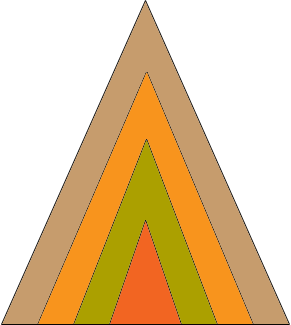SEARCH HOMEMath Central Quandaries & QueriesQuestion from Debbie, a teacher: How do I calculate the perimeter of a triangle if the lengths of two sides are equal and all the angles are known?Debbie,

You can't!The orange, green, yellow and brown triangles in the diagram above each have two sides of equal lengths, the angles of each of the triangles have measures equal to the angles of each of the other triangles and yet the perimeters are different. Do you know the length of a side?

HarleyMath Central is supported by the University of Regina and The Pacific Institute for the Mathematical Sciences.